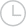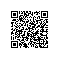# 复杂度分析，使数组中的每个元素等于最大元素 -问答-阿里云开发者社区-阿里云

## 复杂度分析，使数组中的每个元素等于最大元素小六码奴2019-10-03 19:33:0161

for (int i = 0; i < 2*n; i++) { if (i == n) { for (int j = 0; j < i; j++) for (int k = 0; k < i; k++) O(1) } else { for (int j = 0; j < i; j++) O(1) } } 到目前为止，我的想法是：

if语句不能总是为true（可以为log n）。嵌套的for循环内部为n ^ 2。取消 提交回答

• 一码平川MACHEL
2019-10-09 16:28:50

如果不使用if(i == n) {}，则操作数为：

1 + 2 + 3 + 4 + 5 + ... + n*2

= (2n * (2n-1))/2 但是在i==n，操作的数量i与其余的不同，而是i²。因此，最终的操作数为：

((2n * (2n-1))/2) - n + n² 上面的大O表示法是O（n²）

0 0使用钉钉扫一扫加入圈子
+ 订阅